#### How to Calculate Yield in Excel?

People who invest in bonds are always anxious to know the current yield, the yield to maturity and the yield to call of the bonds they purchased. Using Excel, you can develop a bond yield calculator easily with the help of a number of formulas. You just need to enter the inputs like face value, coupon rate, years to maturity etc and Excel will calculate the bond yield and display it for you.

## Prepare data set

Open Excel and save your file as yield.xlsx. Type “Face Value” in A1, “Annual Coupon Rate” in A2, “Annual Required Return” in A3, “Years to Maturity” in A4, “Years to Call” in A5, “Call Premium” in A6, “Payment Frequency” in A7, “Value of Bond” in A9, “Current Yield” in A11, “Yield to Maturity” in A12 and “Yield to Call” in A13. You can format these cells and make them bold. Now your screen will look like this: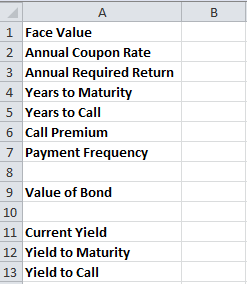Select cells B1 and B9. Right click and go to Format Cells.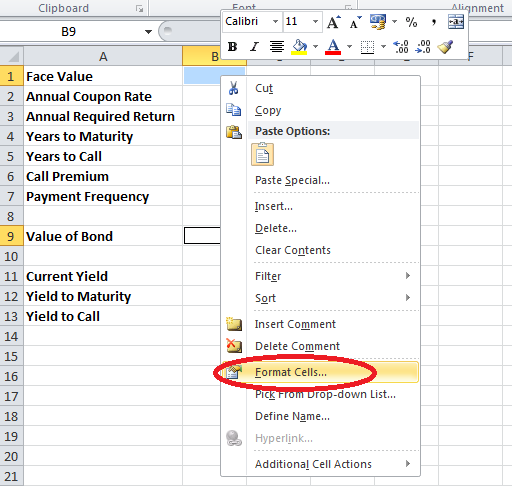You will get a window like this: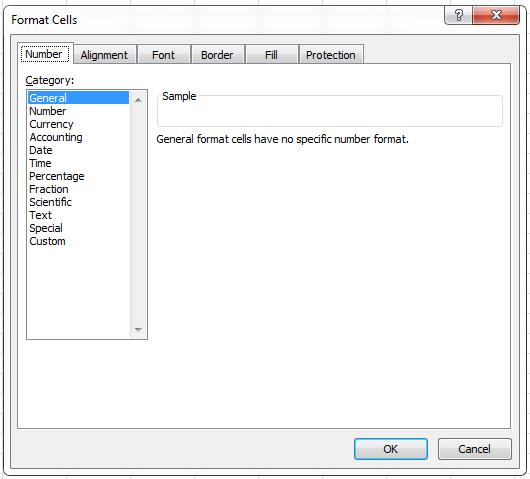From the Category: section on the left hand side, select Currency. Select \$ English (U.S.) from the Symbol: drop down on the right hand side. Now your screen will look like this: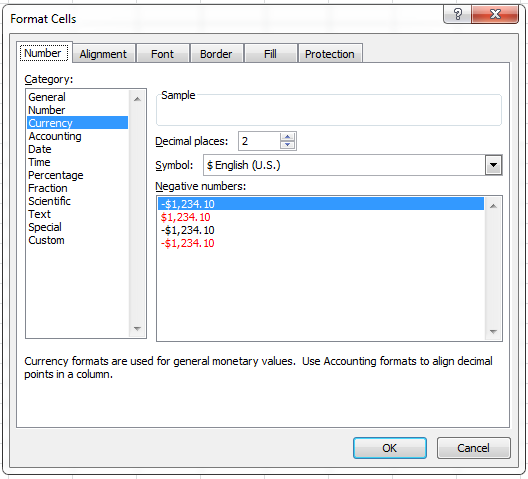Click OK.

Select cells B2, B3, B6, B11, B12 and B13. Right click and go to Format Cells. You will get a new window. From the Category: section on the left hand side, select Percentage. Now your screen will look like this: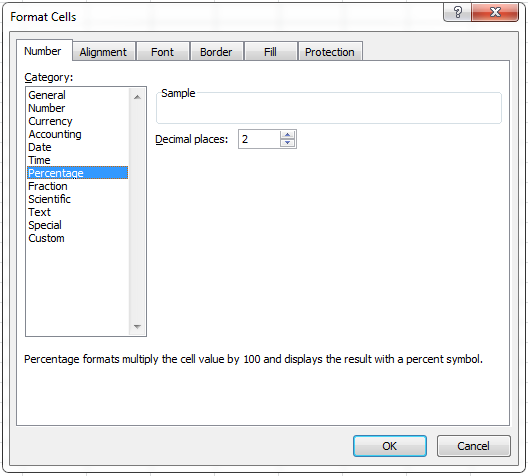Click OK.

Enter some reasonable values in the cells B1, B2, B3, B4 and B7.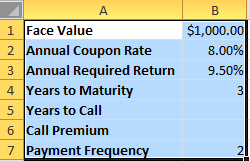## Calculate Yield

To calculate the present value of the bond, click the cell B9. Go to Formulas (main menu) –> Financial (in the Function Library group) and select the PV function.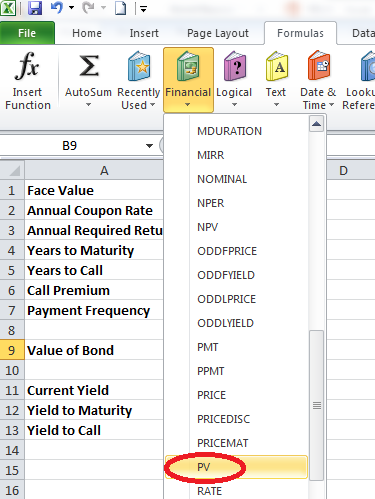You will get a window like this: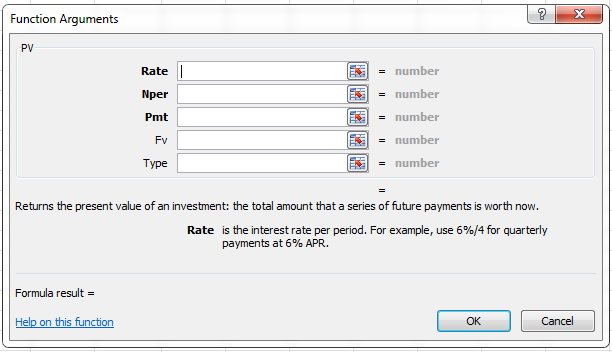In the Rate, Nper, Pmt and Fv textboxes, enter the values B3/B7, B4*B7, B2/B7*B1 and B1 respectively. Now your window like this: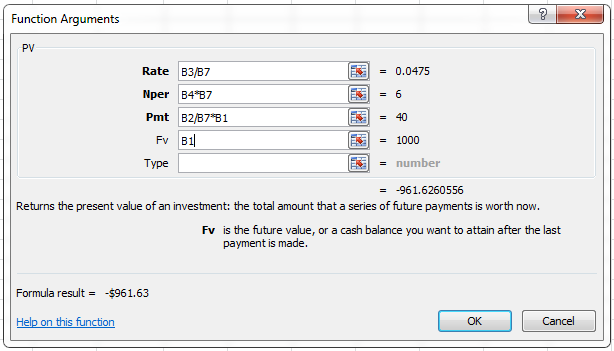Click OK. Now you will get a negative value in the cell B9. Now go to the formula bar and add a – sign just after the = sign like this: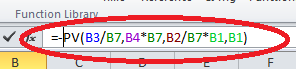To calculate the current yield, click inside the cell B11 and enter the formula “=(B1*B2)/B9” (without double quotes).

To calculate the yield to maturity, click inside the cell B12. Go to Formulas (main menu) –> Financial (in the Function Library group) and select the RATE function. You will get a window like this: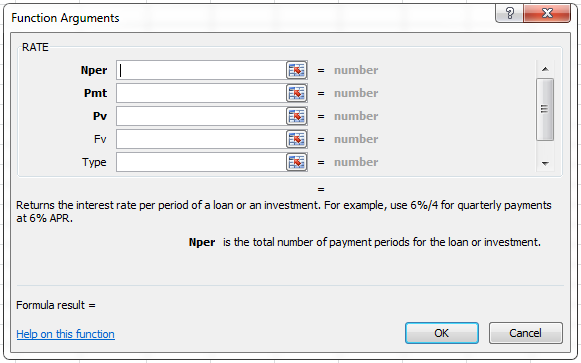In the Rate, Nper, Pmt and Fv textboxes, enter the values B4*B7, B2*B1/B7, -B9 and B1 respectively. Now your window will look like this: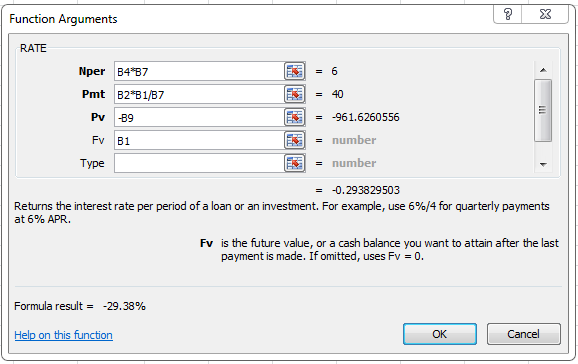Click OK. As this value is for the half year, go to the formula bar and add *B7 at the end of the formula like this: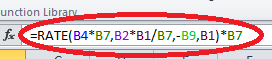Enter reasonable values in the cells B5 and B6 (say 1 and 3).

To calculate the yield to call, click inside the cell B13. Go to Formulas (main menu) –> Financial (in the Function Library group) and select the RATE function. You will get a new window. In the Rate, Nper, Pmt and Fv textboxes, enter the values B5*B7, B2*B1/B7, -B9 and B1*(1+B6) respectively. Now your window will look like this: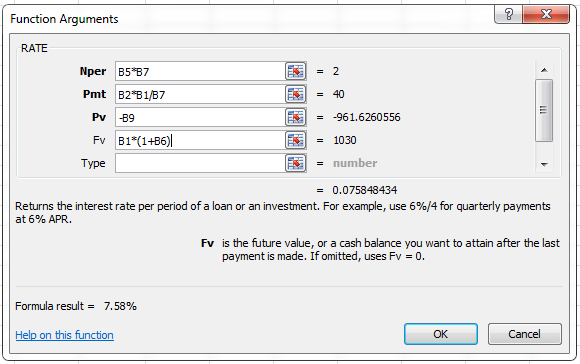Click OK. Go to the formula bar and add *B7 at the end of the formula like this: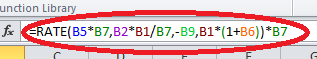Now your bond yield calculator will look like this: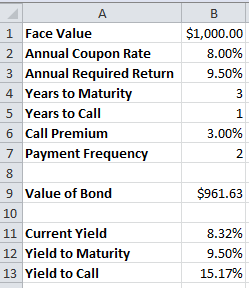By submitting the face value, coupon rate, required return, years to maturity, years to call, call premium and payment frequency, you get the current yield, yield to maturity and yield to call with this bond yield calculator. You can try changing the inputs and observe the difference in the output.

## Calculate Yield using YIELD function

Calculating yield in Excel involves using a financial function called the YIELD function. The YIELD function calculates the yield of a bond, which is the rate of return an investor receives for holding the bond. To use the YIELD function in Excel, follow these steps:

1. Open a new or existing Excel worksheet.
2. Type in the necessary inputs for the bond that you want to calculate yield for. These inputs include the settlement date, maturity date, annual coupon rate, price, and redemption value.
3. In a new cell, type “YIELD(” to start the YIELD function.
4. Type in the necessary arguments for the YIELD function. The arguments include the settlement date, maturity date, annual coupon rate, price, redemption value, frequency, and basis.
5. Press Enter to calculate the yield for the bond.

Here is an example of how to use the YIELD function in Excel:

Assume you have a bond with a settlement date of January 1, 2023, a maturity date of December 31, 2030, an annual coupon rate of 5%, a price of \$950, and a redemption value of \$1,000. The bond pays interest semi-annually and uses the 30/360 day count basis.

To calculate the yield, follow these steps:

1. In a new cell, type “YIELD(“.
2. Type the settlement date in the format “1/1/2023”.
3. Type the maturity date in the format “12/31/2030”.
4. Type the annual coupon rate as a percentage, or “5%” in this example.
5. Type the price of the bond, or “\$950” in this example.
6. Type the redemption value of the bond, or “\$1000” in this example.
7. Type the frequency of the bond payments as “2”, since the bond pays interest semi-annually.
8. Type the day count basis as “1”, since the bond uses the 30/360 day count basis.
9. Press Enter to calculate the yield, which should be approximately 5.83%.

## Calculate Yield using IRR function

There is another alternative method to calculate the yield of a bond in Excel, which involves using the IRR function. The IRR (Internal Rate of Return) function calculates the yield of a bond based on the cash flows generated by the bond. This method is useful when you have the actual cash flows of the bond, rather than just the coupon rate, price, and redemption value.

Here’s how to use the IRR function to calculate the yield of a bond:

1. Open a new or existing Excel worksheet.
2. Type in the cash flows for the bond that you want to calculate yield for. Cash flows include the initial investment, as well as any coupon payments and the redemption value.
3. In a new cell, type “IRR(” to start the IRR function.
4. Select the range of cash flows for the bond, including the initial investment, coupon payments, and redemption value.
5. Press Enter to calculate the yield for the bond.

Here is an example of how to use the IRR function in Excel:

Assume you have a bond with a maturity date of December 31, 2030, an initial investment of \$950, annual coupon payments of \$50, and a redemption value of \$1,000. The cash flows for the bond are as follows:

Year 1: -\$950 (initial investment) Year 2: \$50 (coupon payment) Year 3: \$50 (coupon payment) Year 4: \$50 (coupon payment) Year 5: \$50 (coupon payment) Year 6: \$50 (coupon payment) Year 7: \$50 (coupon payment) Year 8: \$1,050 (redemption value)

To calculate the yield, follow these steps:

1. In a new cell, type “IRR(“.
2. Select the range of cash flows for the bond, including the initial investment, coupon payments, and redemption value (A1:A8 in this example).
3. Press Enter to calculate the yield, which should be approximately 5.83%.

Note that the result of the IRR function is the annualized yield of the bond. If the bond pays interest semi-annually, you will need to divide the result by 2 to get the semi-annual yield.